# MPC5xxx/S32Kxx: CAN / CAN FD bit timing calculation

cancel
Showing results for
Show  only  | Search instead for
Did you mean:

# MPC5xxx/S32Kxx: CAN / CAN FD bit timing calculation

No ratings

## MPC5xxx/S32Kxx: CAN / CAN FD bit timing calculation

This tool simplifies CAN bit timing calculation for CAN modules (FlexCAN, MCAN) available on MPC5xxx and S32Kxxx families.

Enter input parameters into light green cells.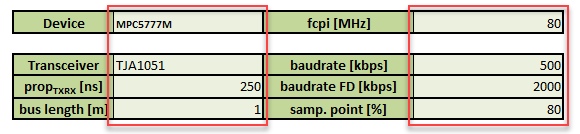Device and Transceiver are selected from pull-down menus.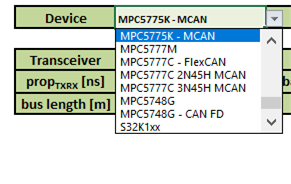By selecting Transceiver, propagation delay parameter is also loaded, but can be simply overwritten by user value.

Rest of parameters can be modified directly upon user needs.

The tool lists possible setting together with register view.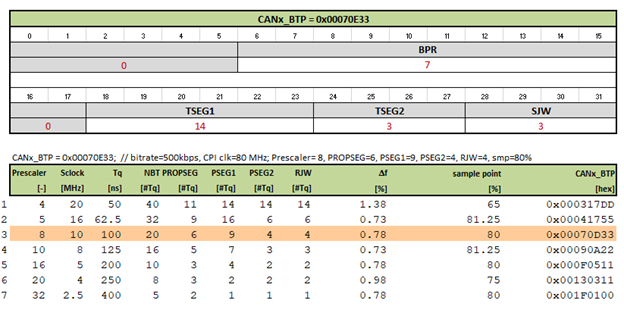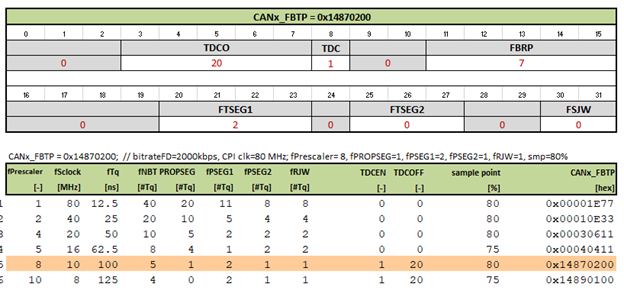A recommended setting is highlighted. Three criteria are used for recommended values selection

- desired sample point

- highest fcpi accuracy

- same prescalers for nominal and data phases, if CAN FD is calculated

For sure other setting can be selected, if needed, by clicking on respective line in list.

Note: Macros have to be enabled!

BR, Petr

Tags (6)Thanks for the tool. I am using MPC5748G with CAN FD supported. I compared this tool and S32DS, they come out with different result with same input parameters.

My test case with following parameters (here we only talk about arbitration rate) :

 Bit rate = 500k bit per second
 Bus length = 4m
 Bus propagation delay = 5 x 10 s/m
 Physical Interface (PCA82C250) transmitter plus receiver propagation delay = 150ns at
85C
 CPI_clock = 40 MHz

 Sample Point 80%

- Step 1:

Physical delay of bus = 4m x 50 ns/m = 200 ns
t[PROP_SEG] = 2*(200+150) = 700 ns
t[PROP_SEG] = 700 ns

- Step 2:

CPI_clock = 40 MHz
Baudrate = 500Kbps
NBT * Prescaler = CPI_clock/Baudrate = 80
8 <= NBT <= 25
1 <= Prescaler <= 256

There can be 4 possibilities:

NBT = 20, Prescaler = 4
NBT = 16, Prescaler = 5
NBT = 10, Prescaler = 8
NBT = 8, Prescaler = 10

We choose NBT = 20 to check.

- Step 3:
PROP_SEG = 7

- Step 4:
2 <= PROP_SEG+PSEG1+2 <=16
2 <= PSEG2+1 <= 8

2 <= 7+PSEG1+2 <= 16

1+ (7+PSEG1+2) + (PSEG2+1) = 20

2 <= 9+PSEG1 <= 16
2 <= PSEG2+1 <= 8

PSEG1+PSEG2 = 9
(1+(7+PSEG1+2))/20 = 80%
(10+PSEG1)/20 = 80%

PSEG1 = 6
PSEG2 = 3

So, one possible combination is PROP_SEG = 7, PSEG1 = 6, PSEG2 = 3, PRESDIV = 3, and this match to the S32DS result.

While when I use the tool in this post, I configured parameters as following:

- Device: MPC5748G-CAN FD

- fcpi: 40

- propTxRX: 150

- bus length: 4

- baudrate: 500

- baudrate FD: 1000 (not used, since we only care about arbi rate in this case)

- samp. point: 80

The result was much different with S32DS's. There was no one parameter suite as expected.

And I am confused with bit timing calculating, it will be appreciated if you can share your experience with following questions:

- in S32DS PE, how PROP_SEG length is calculated? It seems that doesn't get from bus and transceiver delays, and the result different in arbitration phase and data phase (when FD is enabled).

- What's the algorithm you followed for either non-FD or FD bit timing calucation? I only find one document for non-FD, but no FD related document.Hi,

you have mistake in first step. Bus propagation delay is 5 ns/m. So the calculated PROPSEG is different.

Not sure how the bit timing is calculated in the S32DS, no transceiver and bus length is assumed. But if CAN FD is enabled it still uses CTRL1 parameters for calculation, however CBT should be used.

Data phase can be calculated in same manner, for propagation delay just single transceiver prop delay can be assumed.

BR, PetrHi Petr ,

Regarding the sample point that should be configured in the calculator .. If I  have two samples points in my project one for arbitration part which is 81.25  % and another one for CAN-FD data which is 60 % so  which one should I use when I calculate the timing parameters ?

and other thing is there another version of the CAN bit timing Calculation which supports NXPTJA1057GT Transceiver?

Nuha BAHAAHi Petr ,

Regarding the sample point that should be configured in the calculator .. If I  have two samples points in my project one for arbitration part which is 81.25  % and another one for CAN-FD data which is 60 % so  which one should I use when I calculate the timing parameters ?

and other thing is there another version of the CAN bit timing Calculation which supports NXPTJA1057GT Transceiver?

Nuha BAHAAHello Petr,

thanks for providing that useful calculator!

I have a question though about the (recommended) results, namely: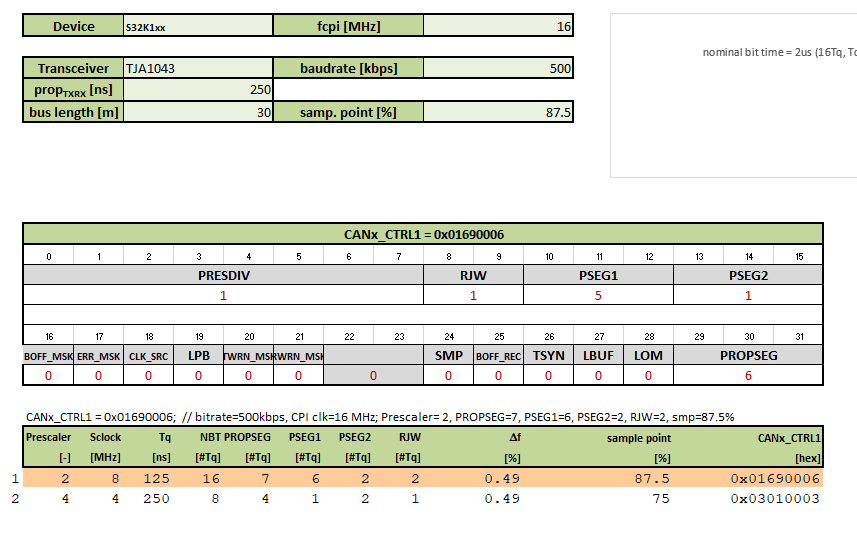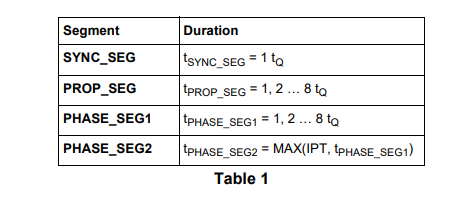In this case, it provides me with a recommended result for which the rule in AN1798 (Table1) tPHASE_SEG2 = MAX(IPT, TPHASE_SEG1) does not seem to be followed.

That makes me wonder, if these parameters can still be used or if the second proposal below with 75% sample point is absolutely required?

Note: RJW would then be 1 in my case (J1939).

Thanks in advance for shedding some light on my problem!

Kind Regards

Michael

Version history
Last update:
‎08-18-2023 03:54 AM
Updated by: